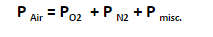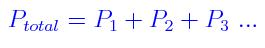# Partial Pressure Formula

The molecules of air frequently bump into the walls of the vessel. These impacts apply an energy on the walls of the vessel which is not anything but termed as pressure. The pressure exerted by the gas is force per unit area. Though as we know, air is a mixture of gases like nitrogen, oxygen, etc. and not a single compound. The pressure that is contributed by every gas is termed its partial pressure. Partial pressure can, therefore, be explained as the pressure applied if that gas by itself had engaged the volume. Therefore, the entire pressure of air will be the summation of partial pressures of oxygen, nitrogen, etc.

The aggregate pressure is, therefore, the summation of partial pressures of the ideal gases. This is articulated by Dalton’s law of partial pressures.where P Air is the aggregate pressure of air, PO2, PN2  are partial pressures of nitrogen, oxygen, etc.

Solved Examples

Problem 1: To a tank containing O2 at 1.0atm and N2 at 2.0atm, we add an anonymous quantity of CO2 up until the total pressure inside the tank is 4.6atm. What is the CO2’s partial pressure?

Dalton’s law states that the addition of CO2 does not upset the partial pressures of the O2 and N2 already existing in the tank. The partial pressure of O2 and N2 remain at 2.0atm, respectively, and their total is 3.0atm. The partial pressure of the added CO2 must be 1.6 atm if the new pressure is 4.6atm, Therefore, when the final pressure is 4.6atm, the partial pressures are4.6atm = 2.0atm + 1.0atm + 1.6atm

Problem 2: What is the partial pressure of the water vapor when a vessel under 2.015atm pressure contains water vapor H2O and nitrogen N2. The partial pressure of N2 is 1.908atm.

Solution:

Given

Total pressure = 2.015atm

Partial pressure of N2 = 1.908atm

Partial pressure of water vapor = ?

At present, we make use of the equationPartial Pressure

P total = PN2 + P water vapor

2.015 = 1.908 + P water vapor

P water vapor = 0.107